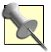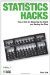# Hack 16. Identify Unexpected RelationshipsIf you want to verify whether a relationship you have observed between two variables is real, you have a variety of statistical tools available. A problem arises, though, when you have measured these variables without much precision, using categorical measurement. The solution is a two-way chi-square test, which, among other things, can be used to make unsubstantiated assumptions about the characteristics of people you have just met.

"Identify Unexpected Outcomes" [Hack #15] used the one-way chi-square test to make police scheduling decisions based on whether equal numbers of crimes were committed at different times of day. That tool works well to solve any analytical problem when:

• The data is at the categorical level of measurement (e.g., gender, political party, ethnicity).

• You want to determine whether there is a greater frequency of scores in certain categories than would be expected by chance.

You face another common analytic problem when you're curious to know whether two categorical variables are related to each other. Relationships between categorical variables can be examined with the handy two-way chi-square test.If two variables are measured at the interval level (many scores are possible along a continuum), the correlation coefficient [Hack #11] is the best tool to use, but it doesn't work well with categorical measurement.

We make assumptions all the time about relationships between these sorts of variables. Many of our common stereotypes about categories of people have implicit hypotheses about these relationships. Here are a few assumptions you might have that imply a relationship between categorical variables:

• Professors are absent-minded.

• Computer programmers play Dungeons and Dragons.

• Adults who collect comics write Statistics Hacks books.

• Professors are absent-minded.

If you meet a computer programmer at a party and you hold this stereotype belief about this type of person, you might assume that she is familiar with 20-sided dice. If you are wrong, though, that might lead to much awkward conversation. It would be nice to know if there really were such relationships between these categorical variables of interest. Calculating a two-way chi-square solves this problem and can verify or cast doubt on these assumptions about people.

## Review of the One-Way Chi-Square

The chi-square test is used in the framework of having certain expectations and seeing whether they are met by the observed data. Statisticians know the size of normal fluctuations in observed frequencies compared to expected frequencies. With this knowledge, they can place a likelihood that any observed deviation from the expected occurred by chance or whether something else is going on. The raw data for these analyses is usually the number of people (the frequency) in each category of some variable.

Here is the general chi-square formula:

S means to add up the things that follow it. The bigger the chi-square, the less likely it is that the outcomes occurred randomly.

While the one-way chi-square analyzes a single categorical variable, two-way chi-squares analyze the relationship between two categorical variables. The process is the same: compare the expected frequencies with actual frequencies for each category or combination of categories. If the differences add up to a big number, then something is going on.

Here is a categorical relationship question that we might like to have answered. It is similar to other issues of stereotype that could be explored:

Are females more likely to be Democrats or Republicans?

##### Conduct preliminary analyses

Look at Table 2-6 for an example of categorical frequency data for, to start, a single categorical variable. This data is fictional, but consistent with published studies, which typically find that Republicans are more likely to be male and that females tend to more commonly identify as Democrats.

##### Table Hypothetical sample of Republicans
MalesFemales
4530

In this random sample of 75 Republicans, 45 are males and 30 are females. That's 60 percent male and 40 percent female. Can we conclude that Republicans in general are more likely to be male than female? If not, we would expect there to be 50 percent males and 50 percent females in our sample.A one-way chi-square could see whether more Republicans are male than female, but that's not the hack we are exploring here.

This isn't our research question, though.

##### Compute the two-way chi-square

Our initial question included only Republicans, so while political party might have seemed like a variable in our first analysis, it was really just a description of the population; it did not vary at all. We can add party to our analysis, though, by adding another categoryDemocrat, for example and recruiting 75 more participants, and suddenly we have data with two variables. Imagine frequency data as shown in Table 2-7.

##### Table Hypothetical sample of voters
PartyMalesFemalesTotals
Republican453075
Democrat344175
Totals7971150

Here we have two categorical variables: party affiliation and sex. We could go ahead and use a one-way analysis to look at either of the two rows by themselves. However, a more typical question would be, "Is there a relationship between party and sex?"Q: "Is there a relationship between party and sex?" A: Reminds me of my freshman year. (Ha! I got a million of 'em. I'll be here all week. Good night, everybody!)

To calculate a standardized measure of the difference between the expected frequencies and the observed frequencies, we use the same formula as with the one-way chi-square. As "Identify Unexpected Outcomes" [Hack #15] demonstrates, we start by totaling up the differences between expected and observed frequencies in each cell (each square of a table).

We do the same with the two-way chi-square. The expected frequency in each cell is equal to the number of people in that cell's row multiplied by the number of people in that cell's column and then divided by the total sample size. Using the data in Table 2-7, the calculations for expected frequencies are shown in Table 2-8.

##### Table Expected frequencies for two-way chi-square analysis
PartyMalesFemales
Republican(75x79) / 150 = 39.5 (75x71) / 150 = 35.5
Democrat(75x79) / 150 = 39.5 (75x71) / 150 = 35.5

Thus, the two-way chi-square calculations look like this:

##### Determine if the chi-square is big enough

Statisticians know that the critical chi-square value for 2x2 tables (like the chi-square we just computed) is 3.84. Chi-square values greater than 3.84 are found by chance about 5 percent of the time or less [Hack #15].

Because our chi-square value was 3.24 and that is less than the key 5 percent value of 3.84, we know that such a fluctuation can occur by chance somewhat greater than 5 percent of the time. We cannot claim statistical significance here, and so we must conclude that though our sample seemed to show a relationship between the two categorical variables of party affiliation and sex, it might have occurred because of chance sampling error. In the population from which the sample was drawn, there might not be any relationship.

#### Why It Works

A two-way chi-square answers this relationship question by looking at differences. This might seem counterintuitive, because most statistics look for differences in order to show, well, a difference, not to show similarities. But here's the thinking:

• If there is no relationship between party and sex, then each sex should be equally split between Republicans and Democrats.

• Also, if there is no relationship, then each party should be equally split between males and females.

• This equal distribution in both directions is what is expected by chance. Large deviations from those expectations suggest that something is going on.

The problem solved with this hack was one of knowing whether a stereotype belief we held was correct. Of course, outside of the real world, in the scientific world, researchers use this tool to explore a wide variety of complex questions.

Two-way chi-squares, sometimes called contingency table analyses, are useful anytime you have two categorical variables and want to see whether there is some dependency of one variable on the other. Our example used variables with just two categories, but similar analyses can be done on variables with many categories. The technical requirements are a bit more complex, but the procedure is the same.

• "Identify Unexpected Outcomes" [Hack #15]Statistics Hacks: Tips & Tools for Measuring the World and Beating the Odds
ISBN: 0596101643
EAN: 2147483647
Year: 2004
Pages: 114
Authors: Bruce Frey

Similar book on Amazon